# PLEASE SOLVE QUESTION 2. 1. Plot absorbance Vs time curves for each tube on the same...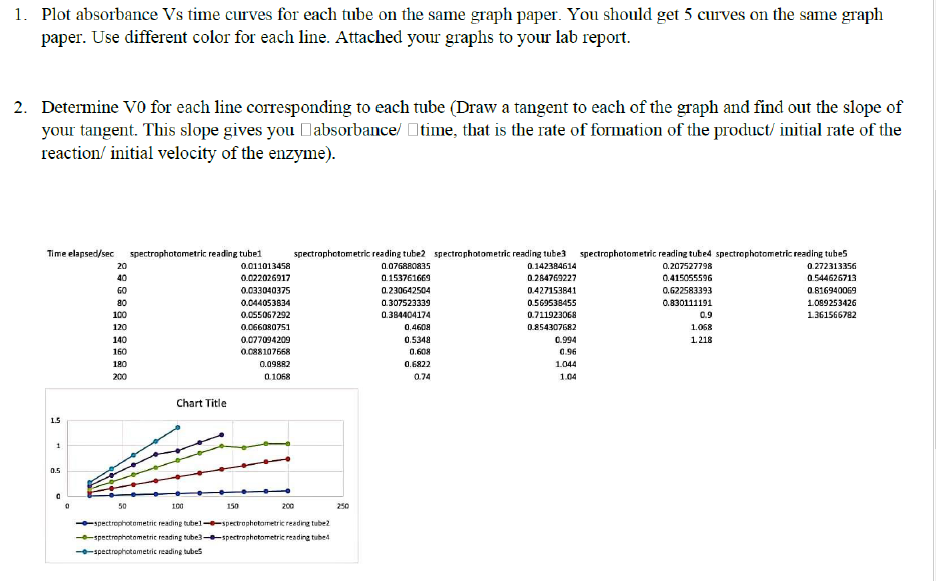Ans 2: As given in the question that the tangent or the slope of each graph gives us the intial rate of the reaction which we have to calculate here. So by using excel i am plotting the 5 graphs are enclosing them below: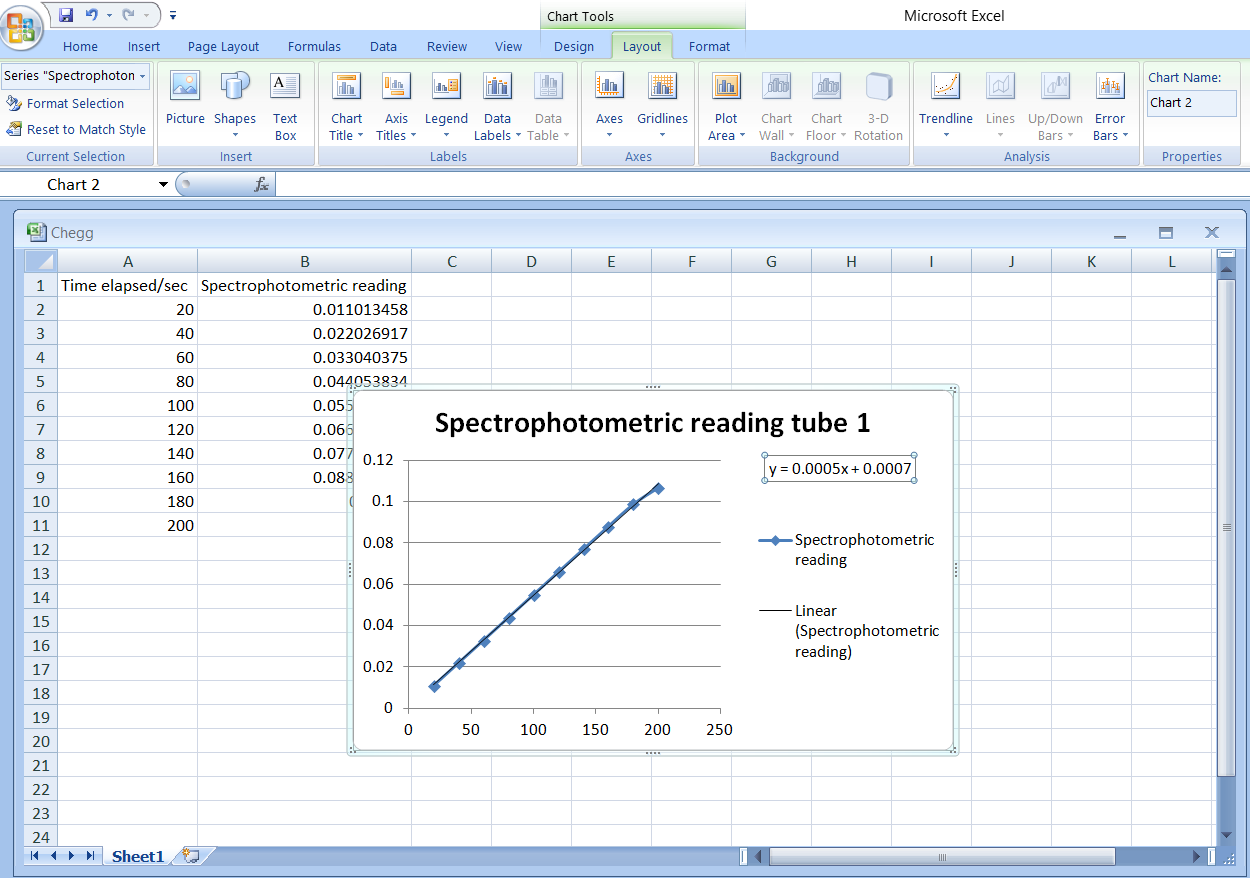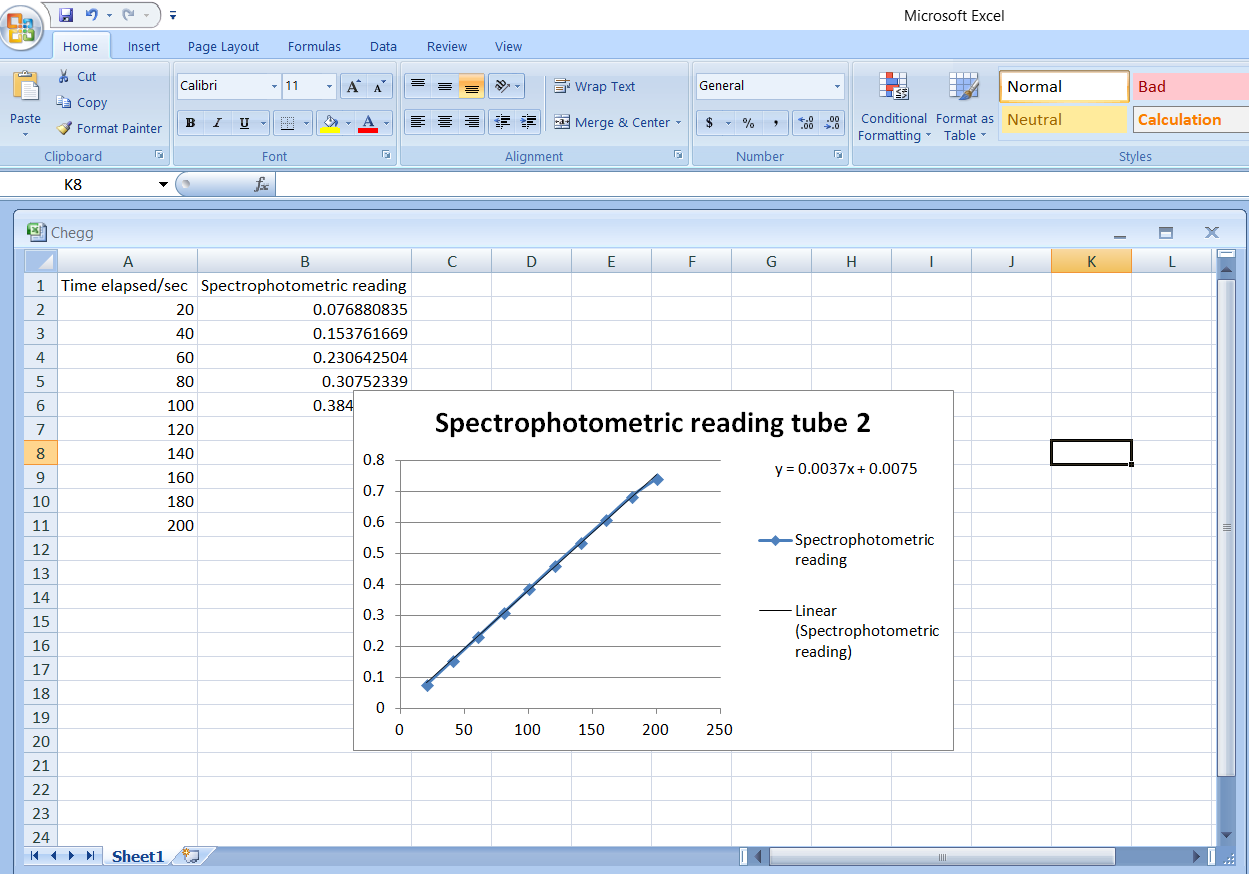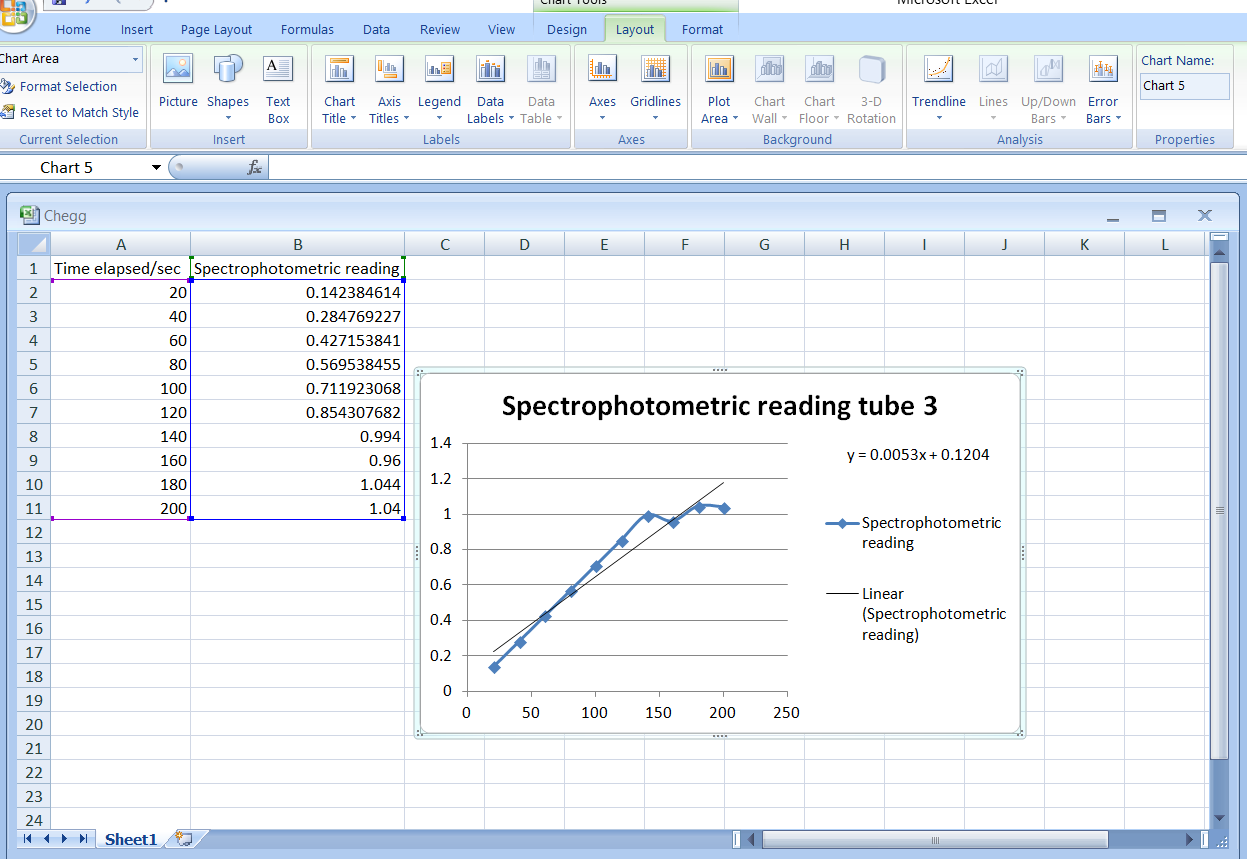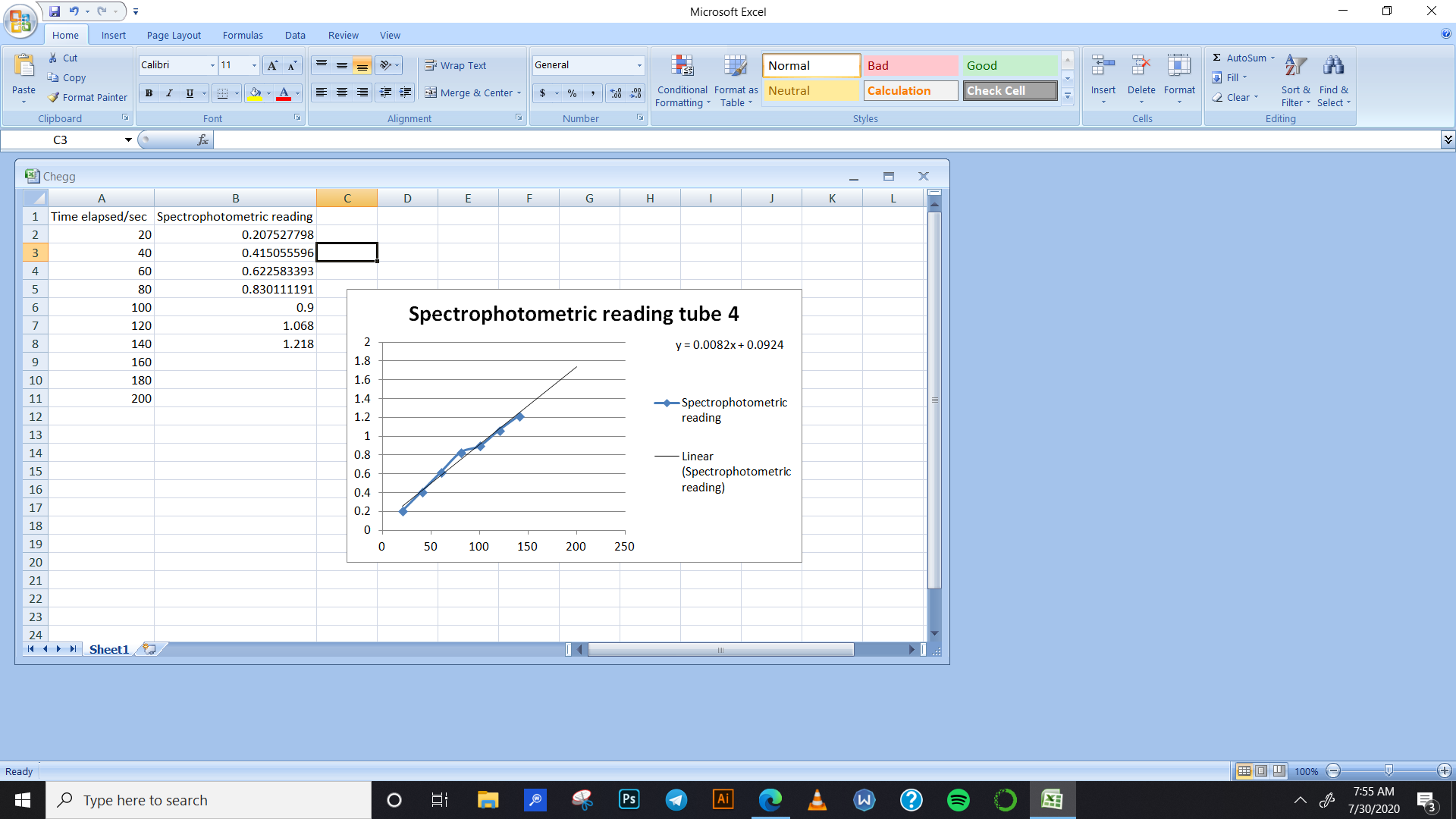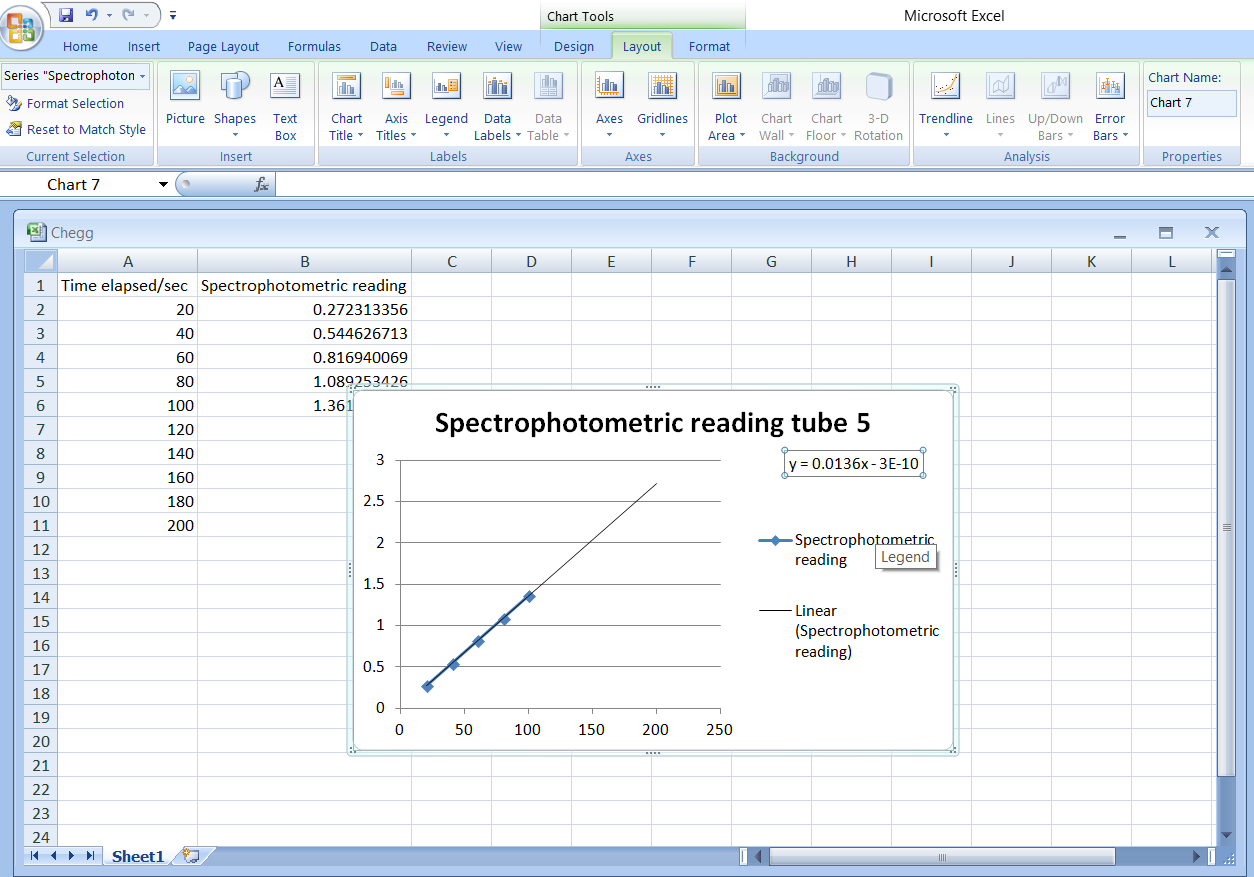So from all the graphs and the respective equations of the slope, we get:

Initial rate of the reaction for tube 1 = 0.0005 concentration/sec.

Initial rate of the reaction for tube 2 = 0.0037 concentration/sec.

Initial rate of the reaction for tube 2 = 0.0053 concentration/sec.

Initial rate of the reaction for tube 2 = 0.0082 concentration/sec.

Initial rate of the reaction for tube 2 = 0.0136 concentration/sec.

##### Add Answer of: PLEASE SOLVE QUESTION 2. 1. Plot absorbance Vs time curves for each tube on the same...
Similar Homework Help Questions
• ### What's the concentration of [FeSCN2+] using limiting reactant theory and equation? For each test tube solution...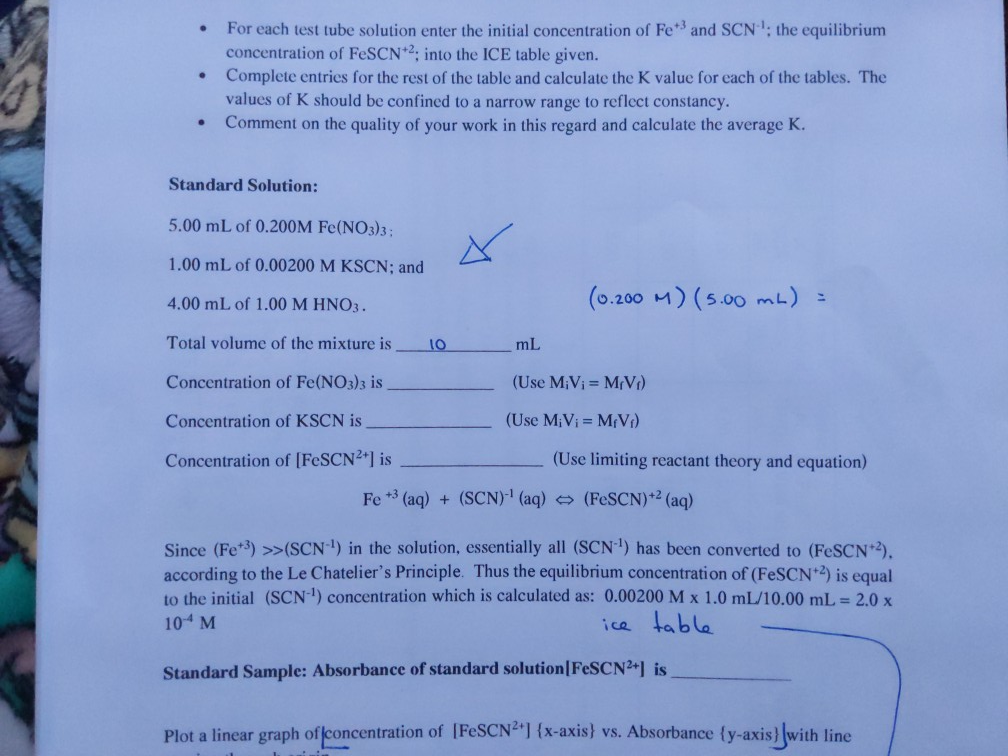What's the concentration of [FeSCN2+] using limiting reactant theory and equation? For each test tube solution enter the initial concentration of Fe+ and SCNthe equilibrium concentration of FeSCN2: into the ICE table given. Complete entries for the rest of the table and calculate the K value for each of the tables. The values of K should be confined to a narrow range to reflect constancy. Comment on the quality of your work in this regard and calculate the average K....

Free Homework App